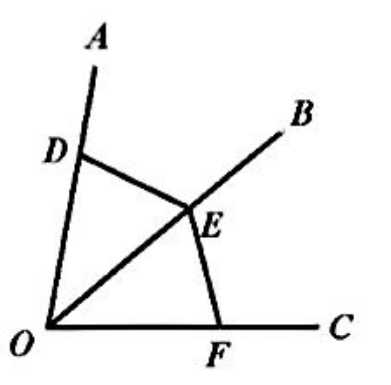$\text{A.}$ $\mathrm{OD}=\mathrm{OE}$ $\text{B.}$ $\mathrm{OE}=\mathrm{OF}$ $\text{C.}$ $\angle \mathrm{ODE}=\angle \mathrm{OED}$ $\text{D.}$ $\angle \mathrm{ODE}=\angle \mathrm{OFE}$
【答案】 D

【解析】 由题意得: $\angle A O B=\angle B O C, O B=O B$, 若使 $\triangle D O E \cong \triangle F O E$, 则需 $O D=O F$ 或除已知外的一组对应角相等即可. 根据选项可知 $\angle O D E=\angle O F E$